# 2. Find the derivative of +e= e. State the solution in the form , where a...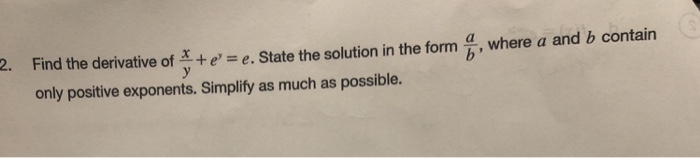2. Find the derivative of +e= e. State the solution in the form , where a and b contain only positive exponents. Simplify as much as possible.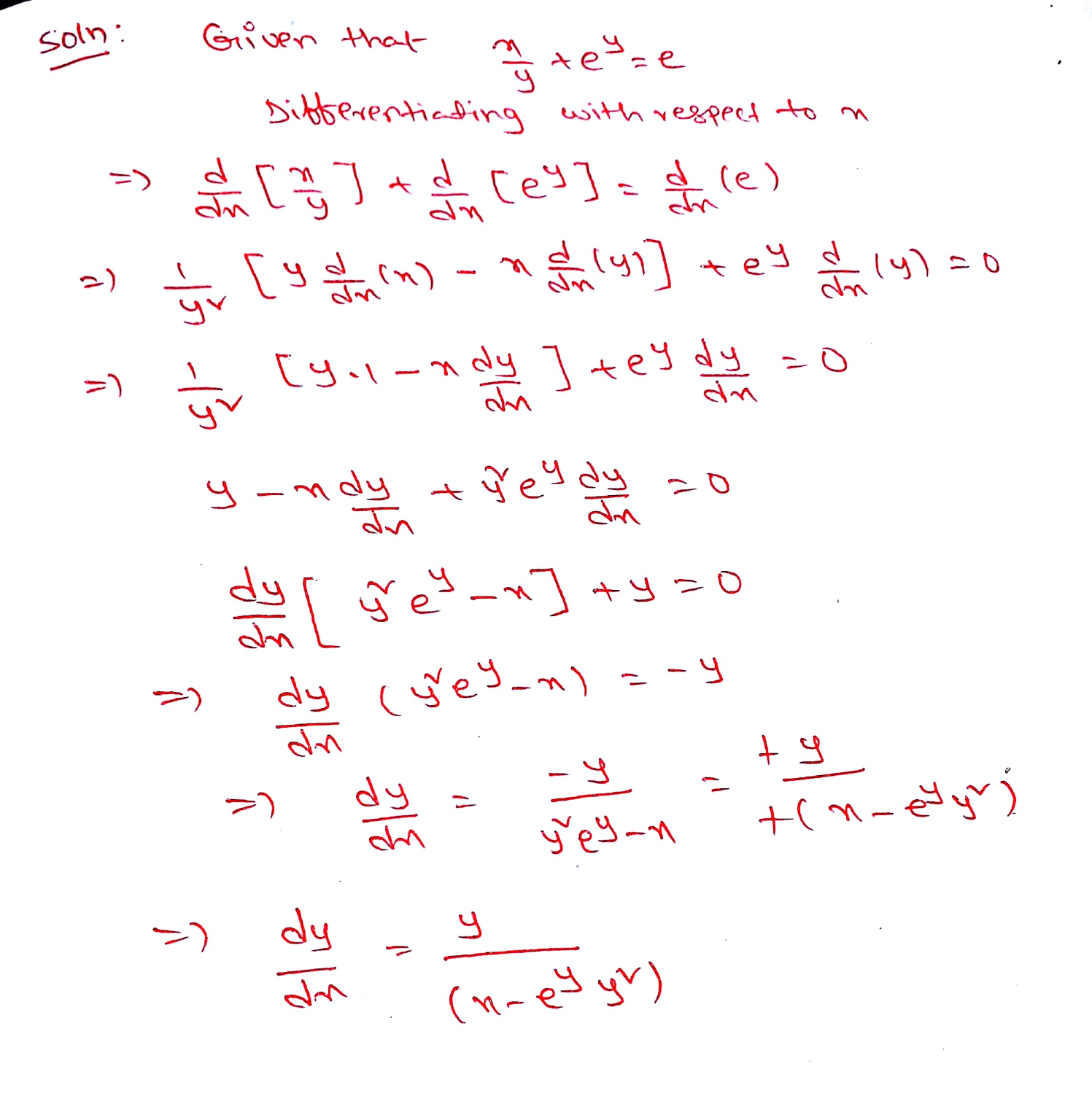##### Add Answer of: 2. Find the derivative of +e= e. State the solution in the form , where a...
Similar Homework Help Questions
• ### find the derivative for the functionFind the derivative for the following function. Simplify if possible to avoid negative exponents.

• ### Find the derivative of the function. Simplify where possible.Find the derivative of the function. Simplify where possible.

• ### Find the derivativeThe answer to this qs is: (Plz do the qs only if you are able to reach to the answer, no incomplete answers plz)Qs: Find the derivative of a function. Simplify where possible:

• ### 2. Using the method of Frobenius, find the general solution about the point i = 0...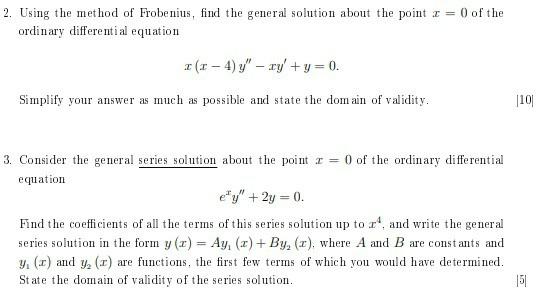2. Using the method of Frobenius, find the general solution about the point i = 0 of the ordinary differential equation 1 (1 - 4) y" - ry' +y = 0. Simplify your answer as much as possible and state the domain of validity. 110 3. Consider the general series solution about the point I = 0 of the ordinary differential equation e'y' + 2y = 0. Find the coefficients of all the terms of this series solution up to...

• ### Find the derivative

Find the derivative of the function. Simplify where possible. y=xcos-1 x - √(1 - x2)

• ### Find the derivative

Find the derivative of the functions. Simplify where possible. y =tan-1(x - √(1 + x2)

• ### D. Find the general solution of the differential equation56-48x + 49y - 42xy dx Give an implicit ...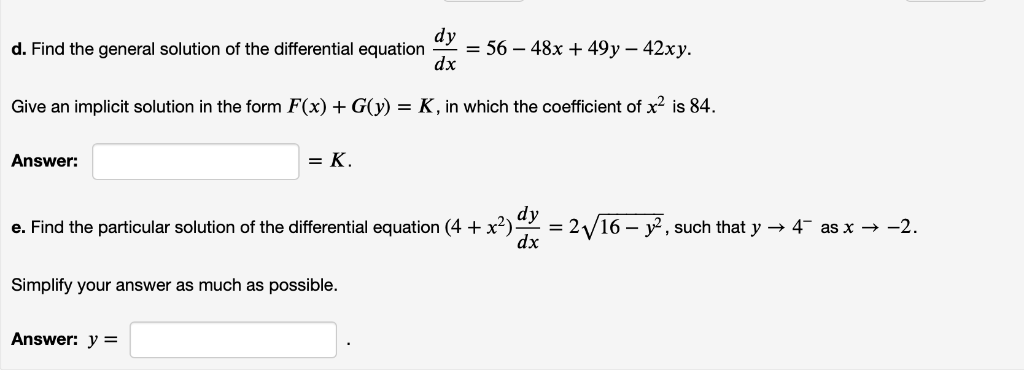d. Find the general solution of the differential equation56-48x + 49y - 42xy dx Give an implicit solution in the form F(x)GK, in which the coefficient of x2 is 84. Answer: =K e. Find the particular solution of the differential equation (4 +x2)으-2V 16-r, such that y-t as x →-2. dx Simplify your answer as much as possible. Answer: y= d. Find the general solution of the differential equation56-48x + 49y - 42xy dx Give an implicit solution in the...

• ### Consider the ODE:3xy"+y' - 2xy = 0. Find the general solution in power series form about...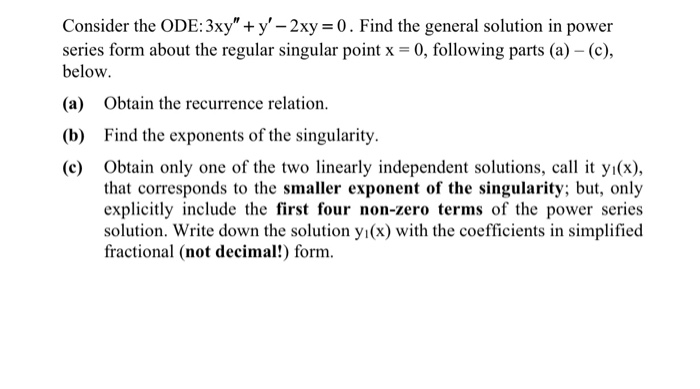Consider the ODE:3xy"+y' - 2xy = 0. Find the general solution in power series form about the regular singular point x = 0, following parts (a) – (c), below. (a) Obtain the recurrence relation. (b) Find the exponents of the singularity. (e) Obtain only one of the two linearly independent solutions, call it y(x), that corresponds to the smaller exponent of the singularity; but, only explicitly include the first four non-zero terms of the power series solution. Write down the...

• ### finding derivativeFind the derivative of the function. Simplify where possible:The answer to this question is: (Ignore the square on top of b)

• ### derivative

Find the derivative of the function. Simplify where possible.y = 9tan^-1 (x - sq.rt of 1+x^2)

Free Homework App